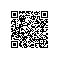# spark-2.2.0-bin-hadoop2.6和spark-1.6.1-bin-hadoop2.6发行包自带案例全面详解（java、python、r和scala）之Basic包下的JavaTC.java（图文详解）

spark-1.6.1-bin-hadoop2.6里Basic包下的JavaTC.java/*
* Licensed to the Apache Software Foundation (ASF) under one or more
* contributor license agreements.  See the NOTICE file distributed with
* this work for additional information regarding copyright ownership.
* The ASF licenses this file to You under the Apache License, Version 2.0
* (the "License"); you may not use this file except in compliance with
* the License.  You may obtain a copy of the License at
*
*    http://www.apache.org/licenses/LICENSE-2.0
*
* Unless required by applicable law or agreed to in writing, software
* distributed under the License is distributed on an "AS IS" BASIS,
* WITHOUT WARRANTIES OR CONDITIONS OF ANY KIND, either express or implied.
* See the License for the specific language governing permissions and
* limitations under the License.
*/

//package org.apache.spark.examples;
package zhouls.bigdata.Basic;

import java.util.ArrayList;
import java.util.HashSet;
import java.util.List;
import java.util.Random;
import java.util.Set;

import scala.Tuple2;

import org.apache.spark.SparkConf;
import org.apache.spark.api.java.JavaPairRDD;
import org.apache.spark.api.java.JavaSparkContext;
import org.apache.spark.api.java.function.PairFunction;

/**
* Transitive closure on a graph, implemented in Java.
* Usage: JavaTC [slices]
*/
public final class JavaTC {

private static final int numEdges = 200;
private static final int numVertices = 100;
private static final Random rand = new Random(42);

static List<Tuple2<Integer, Integer>> generateGraph() {
Set<Tuple2<Integer, Integer>> edges = new HashSet<Tuple2<Integer, Integer>>(numEdges);
while (edges.size() < numEdges) {
int from = rand.nextInt(numVertices);
int to = rand.nextInt(numVertices);
Tuple2<Integer, Integer> e = new Tuple2<Integer, Integer>(from, to);
if (from != to) {
edges.add(e);
}
}
return new ArrayList<Tuple2<Integer, Integer>>(edges);
}

static class ProjectFn implements PairFunction<Tuple2<Integer, Tuple2<Integer, Integer>>,
Integer, Integer> {
static final ProjectFn INSTANCE = new ProjectFn();

@Override
public Tuple2<Integer, Integer> call(Tuple2<Integer, Tuple2<Integer, Integer>> triple) {
return new Tuple2<Integer, Integer>(triple._2()._2(), triple._2()._1());
}
}

public static void main(String[] args) {
SparkConf sparkConf = new SparkConf().setAppName("JavaHdfsLR").setMaster("local");
JavaSparkContext sc = new JavaSparkContext(sparkConf);
Integer slices = (args.length > 0) ? Integer.parseInt(args): 2;
JavaPairRDD<Integer, Integer> tc = sc.parallelizePairs(generateGraph(), slices).cache();

// Linear transitive closure: each round grows paths by one edge,
// by joining the graph's edges with the already-discovered paths.
// e.g. join the path (y, z) from the TC with the edge (x, y) from
// the graph to obtain the path (x, z).

// Because join() joins on keys, the edges are stored in reversed order.
JavaPairRDD<Integer, Integer> edges = tc.mapToPair(
new PairFunction<Tuple2<Integer, Integer>, Integer, Integer>() {
@Override
public Tuple2<Integer, Integer> call(Tuple2<Integer, Integer> e) {
return new Tuple2<Integer, Integer>(e._2(), e._1());
}
});

long oldCount;
long nextCount = tc.count();
do {
oldCount = nextCount;
// Perform the join, obtaining an RDD of (y, (z, x)) pairs,
// then project the result to obtain the new (x, z) paths.
tc = tc.union(tc.join(edges).mapToPair(ProjectFn.INSTANCE)).distinct().cache();
nextCount = tc.count();
} while (nextCount != oldCount);

System.out.println("TC has " + tc.count() + " edges.");
sc.stop();
}
}

spark-2.2.0-bin-hadoop2.6里Basic包下的JavaTC.java/*
* Licensed to the Apache Software Foundation (ASF) under one or more
* contributor license agreements.  See the NOTICE file distributed with
* this work for additional information regarding copyright ownership.
* The ASF licenses this file to You under the Apache License, Version 2.0
* (the "License"); you may not use this file except in compliance with
* the License.  You may obtain a copy of the License at
*
*    http://www.apache.org/licenses/LICENSE-2.0
*
* Unless required by applicable law or agreed to in writing, software
* distributed under the License is distributed on an "AS IS" BASIS,
* WITHOUT WARRANTIES OR CONDITIONS OF ANY KIND, either express or implied.
* See the License for the specific language governing permissions and
* limitations under the License.
*/

//package org.apache.spark.examples;
package zhouls.bigdata.Basic;

import java.util.ArrayList;
import java.util.HashSet;
import java.util.List;
import java.util.Random;
import java.util.Set;

import scala.Tuple2;

import org.apache.spark.api.java.JavaPairRDD;
import org.apache.spark.api.java.JavaSparkContext;
import org.apache.spark.api.java.function.PairFunction;
import org.apache.spark.sql.SparkSession;

/**
* Transitive closure on a graph, implemented in Java.
* Usage: JavaTC [partitions]
*/
public final class JavaTC {

private static final int numEdges = 200;
private static final int numVertices = 100;
private static final Random rand = new Random(42);

static List<Tuple2<Integer, Integer>> generateGraph() {
Set<Tuple2<Integer, Integer>> edges = new HashSet<>(numEdges);
while (edges.size() < numEdges) {
int from = rand.nextInt(numVertices);
int to = rand.nextInt(numVertices);
Tuple2<Integer, Integer> e = new Tuple2<>(from, to);
if (from != to) {
edges.add(e);
}
}
return new ArrayList<>(edges);
}

static class ProjectFn implements PairFunction<Tuple2<Integer, Tuple2<Integer, Integer>>,
Integer, Integer> {
static final ProjectFn INSTANCE = new ProjectFn();

@Override
public Tuple2<Integer, Integer> call(Tuple2<Integer, Tuple2<Integer, Integer>> triple) {
return new Tuple2<>(triple._2()._2(), triple._2()._1());
}
}

public static void main(String[] args) {
SparkSession spark = SparkSession
.builder()
.master("local")
.appName("JavaTC")
.getOrCreate();

JavaSparkContext jsc = new JavaSparkContext(spark.sparkContext());

Integer slices = (args.length > 0) ? Integer.parseInt(args): 2;
JavaPairRDD<Integer, Integer> tc = jsc.parallelizePairs(generateGraph(), slices).cache();

// Linear transitive closure: each round grows paths by one edge,
// by joining the graph's edges with the already-discovered paths.
// e.g. join the path (y, z) from the TC with the edge (x, y) from
// the graph to obtain the path (x, z).

// Because join() joins on keys, the edges are stored in reversed order.
JavaPairRDD<Integer, Integer> edges = tc.mapToPair(e -> new Tuple2<>(e._2(), e._1()));

long oldCount;
long nextCount = tc.count();
do {
oldCount = nextCount;
// Perform the join, obtaining an RDD of (y, (z, x)) pairs,
// then project the result to obtain the new (x, z) paths.
tc = tc.union(tc.join(edges).mapToPair(ProjectFn.INSTANCE)).distinct().cache();
nextCount = tc.count();
} while (nextCount != oldCount);

System.out.println("TC has " + tc.count() + " edges.");
spark.stop();
}
}使用钉钉扫一扫加入圈子
+ 订阅